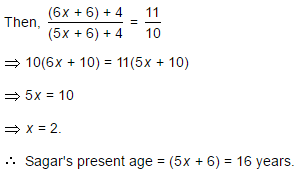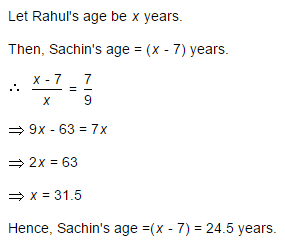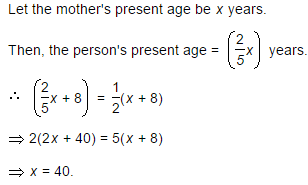CLAT  >  Test: Problems On Ages - 3

# Test: Problems On Ages - 3

Test Description

## 10 Questions MCQ Test Quantitative Techniques for CLAT | Test: Problems On Ages - 3

Test: Problems On Ages - 3 for CLAT 2023 is part of Quantitative Techniques for CLAT preparation. The Test: Problems On Ages - 3 questions and answers have been prepared according to the CLAT exam syllabus.The Test: Problems On Ages - 3 MCQs are made for CLAT 2023 Exam. Find important definitions, questions, notes, meanings, examples, exercises, MCQs and online tests for Test: Problems On Ages - 3 below.
Solutions of Test: Problems On Ages - 3 questions in English are available as part of our Quantitative Techniques for CLAT for CLAT & Test: Problems On Ages - 3 solutions in Hindi for Quantitative Techniques for CLAT course. Download more important topics, notes, lectures and mock test series for CLAT Exam by signing up for free. Attempt Test: Problems On Ages - 3 | 10 questions in 20 minutes | Mock test for CLAT preparation | Free important questions MCQ to study Quantitative Techniques for CLAT for CLAT Exam | Download free PDF with solutions
 1 Crore+ students have signed up on EduRev. Have you?
Test: Problems On Ages - 3 - Question 1

### A man is 24 years older than his son. In two years, his age will be twice the age of his son. The present age of his son is:

Detailed Solution for Test: Problems On Ages - 3 - Question 1

Let the son's present age be x years. Then, man's present age = (x + 24) years.(x + 24) + 2 = 2(x + 2)x + 26 = 2x + 4x = 22.

Test: Problems On Ages - 3 - Question 2

### Six years ago, the ratio of the ages of Kunal and Sagar was 6 : 5. Four years hence, the ratio of their ages will be 11 : 10. What is Sagar's age at present?

Detailed Solution for Test: Problems On Ages - 3 - Question 2

Let the ages of Kunal and Sagar 6 years ago be 6x and 5x years respectively.Test: Problems On Ages - 3 - Question 3

### The sum of the present ages of a father and his son is 60 years. Six years ago, father's age was five times the age of the son. After 6 years, son's age will be:

Detailed Solution for Test: Problems On Ages - 3 - Question 3

Let the present ages of son and father be x and (60 -x) years respectively.

Then, (60 - x) - 6 = 5(x - 6)54 - x = 5x - 306x = 84x = 14.Son's age after 6 years = (x+ 6) = 20 years..

Test: Problems On Ages - 3 - Question 4

At present, the ratio between the ages of Arun and Deepak is 4 : 3. After 6 years, Arun's age will be 26 years. What is the age of Deepak at present ?

Detailed Solution for Test: Problems On Ages - 3 - Question 4

Let the present ages of Arun and Deepak be 4x years and 3x years respectively. Then,

4x + 6 = 264x = 20

x = 5.Deepak's age = 3x = 15 years.

Test: Problems On Ages - 3 - Question 5

Sachin is younger than Rahul by 7 years. If their ages are in the respective ratio of 7 : 9, how old is Sachin?

Detailed Solution for Test: Problems On Ages - 3 - Question 5Test: Problems On Ages - 3 - Question 6

The present ages of three persons in proportions 4 : 7 : 9. Eight years ago, the sum of their ages was 56. Find their present ages (in years).

Detailed Solution for Test: Problems On Ages - 3 - Question 6

Let their present ages be 4x, 7x and 9x years respectively.

Then, (4x - 8) + (7x - 8) + (9x - 8) = 5620x = 80x = 4.Their present ages are 4x = 16 years, 7x = 28 years and 9x = 36 years respectively.

Test: Problems On Ages - 3 - Question 7

Ayesha's father was 38 years of age when she was born while her mother was 36 years old when her brother four years younger to her was born. What is the difference between the ages of her parents?

Detailed Solution for Test: Problems On Ages - 3 - Question 7

Mother's age when Ayesha's brother was born = 36 years.

Father's age when Ayesha's brother was born = (38 + 4) years = 42 years.Required difference = (42 - 36) years = 6 years.

Test: Problems On Ages - 3 - Question 8

A person's present age is two-fifth of the age of his mother. After 8 years, he will be one-half of the age of his mother. How old is the mother at present?

Detailed Solution for Test: Problems On Ages - 3 - Question 8Test: Problems On Ages - 3 - Question 9

Q is as much younger than R as he is older than T. If the sum of the ages of R and T is 50 years, what is definitely the difference between R and Q's age?

Detailed Solution for Test: Problems On Ages - 3 - Question 9

Given that:

1. The difference of age b/w R and Q = The difference of age b/w Q and T.

2. Sum of age of R and T is 50 i.e. (R + T) = 50.

Question: R - Q = ?.

Explanation:

R - Q = Q - T

(R + T) = 2Q

Now given that, (R + T) = 50

So, 50 = 2Q and therefore Q = 25.

Question is (R - Q) = ?

Here we know the value(age) of Q (25), but we don't know the age of R.

Therefore, (R-Q) cannot be determined.

Test: Problems On Ages - 3 - Question 10

The age of father 10 years ago was thrice the age of his son. Ten years hence, father's age will be twice that of his son. The ratio of their present ages is:

Detailed Solution for Test: Problems On Ages - 3 - Question 10

Let the ages of father and son 10 years ago be 3x and x years respectively.

Then, (3x + 10) + 10 = 2[(x + 10) + 10]3x + 20 = 2x + 40x = 20.Required ratio = (3x + 10) : (x + 10) = 70 : 30 = 7 : 3.

## Quantitative Techniques for CLAT

56 videos|35 docs|91 tests
 Use Code STAYHOME200 and get INR 200 additional OFF Use Coupon Code
Information about Test: Problems On Ages - 3 Page
In this test you can find the Exam questions for Test: Problems On Ages - 3 solved & explained in the simplest way possible. Besides giving Questions and answers for Test: Problems On Ages - 3, EduRev gives you an ample number of Online tests for practice

## Quantitative Techniques for CLAT

56 videos|35 docs|91 tests